# Chapter 2 : Fractions and Decimals

### Topics covered in this snack-sized chapter:

#### Fractions arrow_upward

• A Fraction is a part of an entire object.
• A fractional number is part of a whole number.
• A fraction has a numerator and a denominator.
•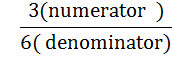• The term on top is called the numerator, and the term on bottom is called the denominator.
• Examples:
•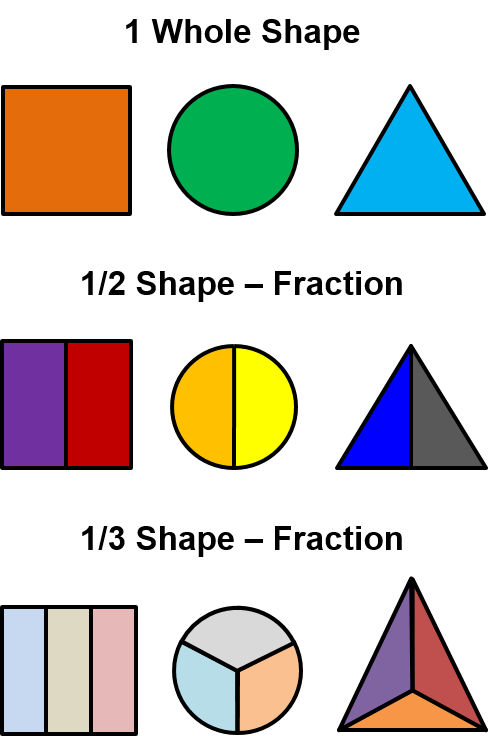#### Types of Fractions arrow_upward

• There are three major types of fractions:

•  Proper Fractions If the numerator of the fraction is less than the denominator, the fraction is said to be proper. All proper fractions are less than 1. Examples: 2/3, 1/3, 5/25. Improper Fractions If the numerator of the fraction is greater than the denominator, the fraction is said to be improper. Examples: 3/2, 10/3, 65/25. Mixed Fractions A fractional number that contains a combination of whole number and a fraction is said to be a mixed fraction. Example: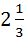On dividing two numbers, if a remainder arrives, the result of division can be represented as a mixed fraction of the form: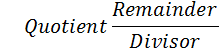Example: 11/3 gives the quotient 3, remainder 2 and divisor 3. So, the mixed form will be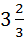.

#### Addition and Subtraction of Fraction arrow_upward

• If the denominators of both fractions are same, add only numerator value. Take the denominator common for both addition and subtraction.
• If the denominators of both fractions are not same then make the denominators common before addition or subtraction.
•

• Example: Solve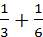.
• Solution: The bottom numbers are different. So, we need to make them same before proceeding further.
• The number "6" is twice as big as "3", so to make the bottom numbers same we will multiply the top and bottom of the first fraction by 2, like this:
•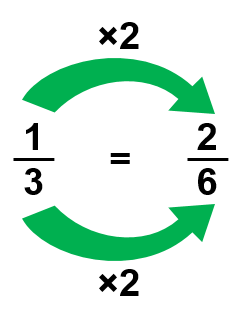• Now the fractions have the same denominator ("6"), and look like this:
•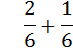• The denominator of both the fractions is same so add only the numerators, taking the denominator common.
•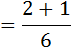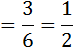#### Multiplying Fractions arrow_upward

• In order to multiply two fractions, multiply the numerators and denominators separately to get the new fraction.
• Example:
•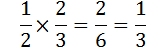#### Dividing Fractions arrow_upward

• The mechanism of division is as follows:
•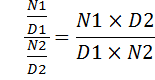• Remember, a ÷ b means a × (1/b).
• And a ÷ (b/c) means a × (c/b).
• Example:
•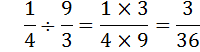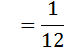#### Decimals arrow_upward

• Decimals are fractions in disguise.
• Any number can be written in the decimal system using only the ten basic symbols.
• 0, 1, 2, 3, 4, 5, 6, 7, 8, and 9
• Decimal positions are shown below:

•  Thousandths Hundredths Tenths 0.001 0.01 0.1

###### Left of the Decimal Point:

• One place to the left of the decimal point is referred to as the unit place.
• Two places to the left of the decimal point is the tens place.
• Three places to the left is the hundreds place, four places is the thousands place, and so on.

• ###### Right of the Decimal Point:

• One place to the right of the decimal point is referred to as tenths place.
• Two places to the right of the decimal point is the hundredths place.
• Three places right is the thousandths place, four places right the ten thousandths place, and so on.

• Write the decimals in a column with the decimal points vertically aligned.
• Add enough zeros to the right of the decimal point so that every number has an entry in each column to the right of the decimal point.
• Add the numbers in the same way as whole numbers.
• Place a decimal point in the sum such that it is directly beneath the decimal points.
• Example: Add 23.143, 3.2756 and 11.48?
• Solution:
•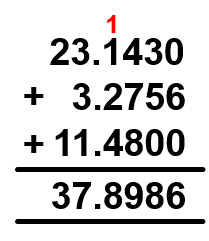#### Subtracting Decimals arrow_upward

• Write the number that is being subtracted from.
• Write the number that is being subtracted below the first number so that the decimal point of the bottom number is directly below and lined up with the top decimal point.
• Add zeros to the right side of the decimal with fewer decimal places so that each decimal has the same number of decimal places.
• Subtract the bottom number from the top number.
• Example: Subtract 3.2756 from 11.48?
• Solution:
•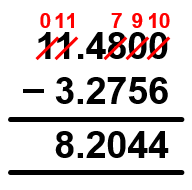#### Multiplying Decimals arrow_upward

• Line up the numbers on the right - do not align the decimal points.
• Starting on the right, multiply each digit in the top number by each digit in the bottom number, just as with whole numbers.
• Place the decimal point in the answer by starting at the right and moving a number of places equal to the sum of the decimal places in both numbers multiplied.
• Example: Multiply 3.77 and 2.8?
• Solution:
•• Now, 3.77 have 2 decimal places and 2.8 has 1 decimal place.
• The sum of decimal places: 2 + 1 = 3
• In the product, we mark decimal point by counting 3 places from right to left.
• Hence, 3.77 × 2.8 = 10.556

• #### Dividing Decimals arrow_upward

• If the divisor is not a whole number:
• Move the decimal point in the divisor all the way to the right (to make it a whole number).
• Move the decimal point in the dividend the same number of places.
• Divide as usual.
• If the divisor doesn’t go into the dividend evenly, add zeroes to the right of the last digit in the dividend and keep dividing until it comes out evenly or a repeating pattern shows up.
• Put the decimal point in the quotient exactly above where it occurs in the dividend.
• Example: Find the quotient: 315.3 ÷ 0.3?
• Solution: We first remove the decimal point from the denominator by multiplying both numerator and denominator with 10, 100 or 1000 accordingly to remove decimal point from the divisor.
• Then we perform the operation of division as usual.
•     315.3 ÷ 0.3

• Since the divisor is a 1 place decimal, so we multiply dividend by 10 to convert into a whole number.
•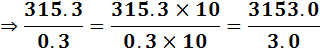• Thus, 315.3 ÷ 0.3 = 1051

• #### Thank You from Kimavi arrow_upward

• Please email us at Admin@Kimavi.com and help us improve this tutorial.